Quadratic formula calculator solves the second-order polynomial equation. It finds the imaginary or real values of the unknown variable.

Give Us Feedback

The quadratic equation solver simplifies the quadratic equation in detail. The calculator is capable of finding both imaginary and real roots.

The steps are explained for user comprehension. You can use it multiple times without any charge or registration.

## What is the Quadratic formula?

The quadratic formula is one of the three main methods to solve a polynomial equation with an order 2. The formula is easy to memorize and use.

A second-degree polynomial is ax2 + bx + c. The arrangement is descending in terms of exponents

Since this equation is known as the quadratic equation, its solving formula is also known as the quadratic formula. The quadratic formula is: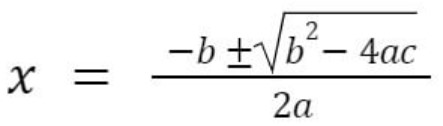The value of the variables is identified from the quadratic equation. Variable a is the coefficient of the x2 known as the quadratic and variable b is the coefficient of the x and is called the linear coefficient.

And c is the constant of the polynomial equation. The (b2- 4ac) part of the formula is called the Determinant. It determines the nature of the roots. The information obtained from its values is: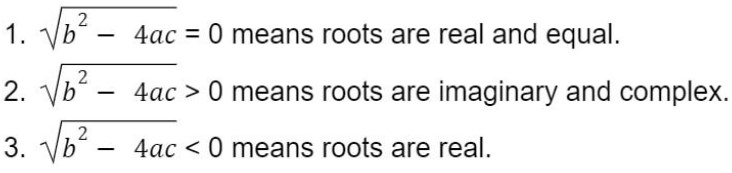When the determinant is equal to zero, you get only one root. This means that the second root is also equal to this one.

Find the determinants of matrices through the determinant calculator

## How to solve the Quadratic equation?

The process of solving the quadratic equation using the formula will be explained ahead. But if you want to try the fast mean for solving the quadratic equation, use the quadratic formula solver.

The process of solving includes the following 5 steps.

• ### Standard form

To apply the quadratic formula correctly, you need to convert the polynomial expression to the standard quadratic form. You can use the vertex form to quadratic form converter for this step.

The standard form, as said before, has the descending degree syntax i.e ax2 + bx + c.

• ### Form an equation

Make the quadratic expression an equation by taking it equal to zero. For example ax2 + bx + c = 0.

• ### Find the Coefficients

Now, you have to identify the values of the coefficients of x2, x, and the constant value. E.g a, b, and c.

• ### Put the values

For the fourth step, you enter the identified values in the quadratic formula.

• ### Solve

Finally, simplify the formula for the roots. If you find the determinant separately first, it can give you an idea of the nature of the roots in advance and hence verify your answer.

These are the steps you need to carry out. For even more understanding, see the example below.

Example:

Solve for the values of x.

6 - 4x + 3x2

Solution:

Step 1: Arrange the expression in the standard form.

3x2 - 4x + 6

Step 2: Make an equation.

3x2 - 4x + 6 = 0

Step 3: Identify the coefficients.

a = 3

b = -4

c = 6

Step 4: Plug the values in the formula.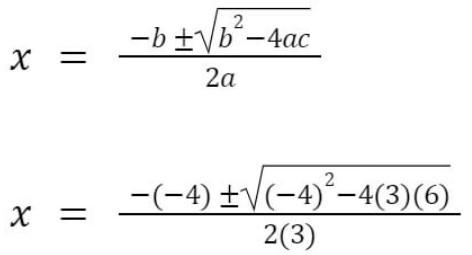Step 5: Simplify.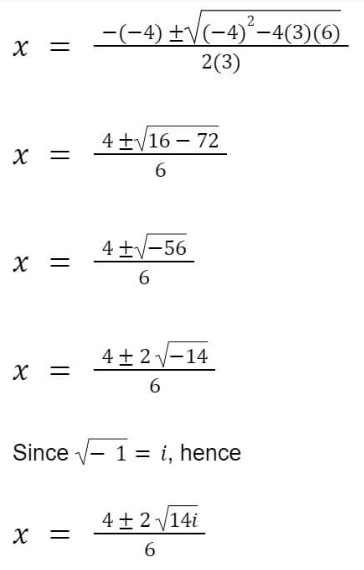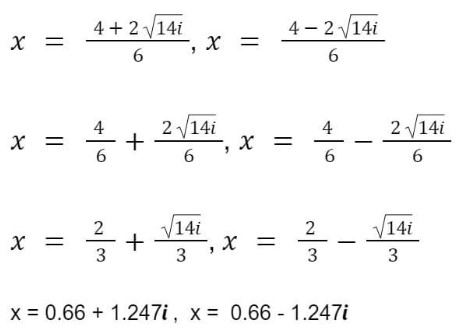### Math Tools